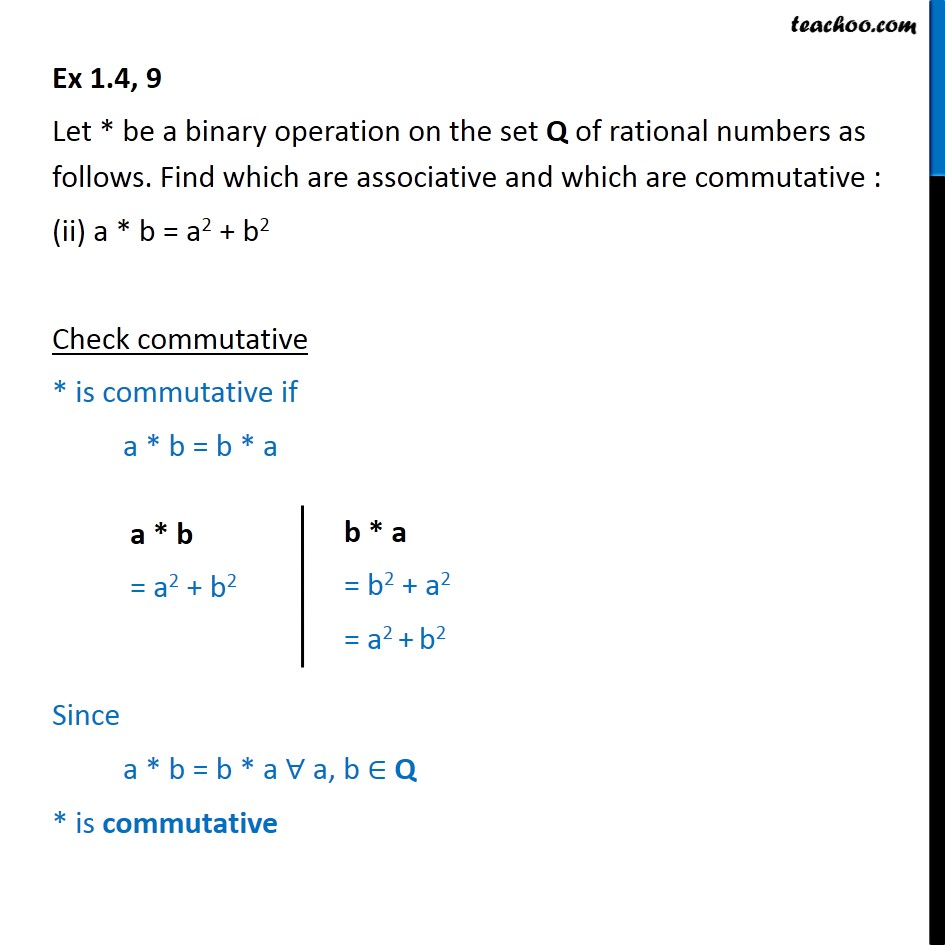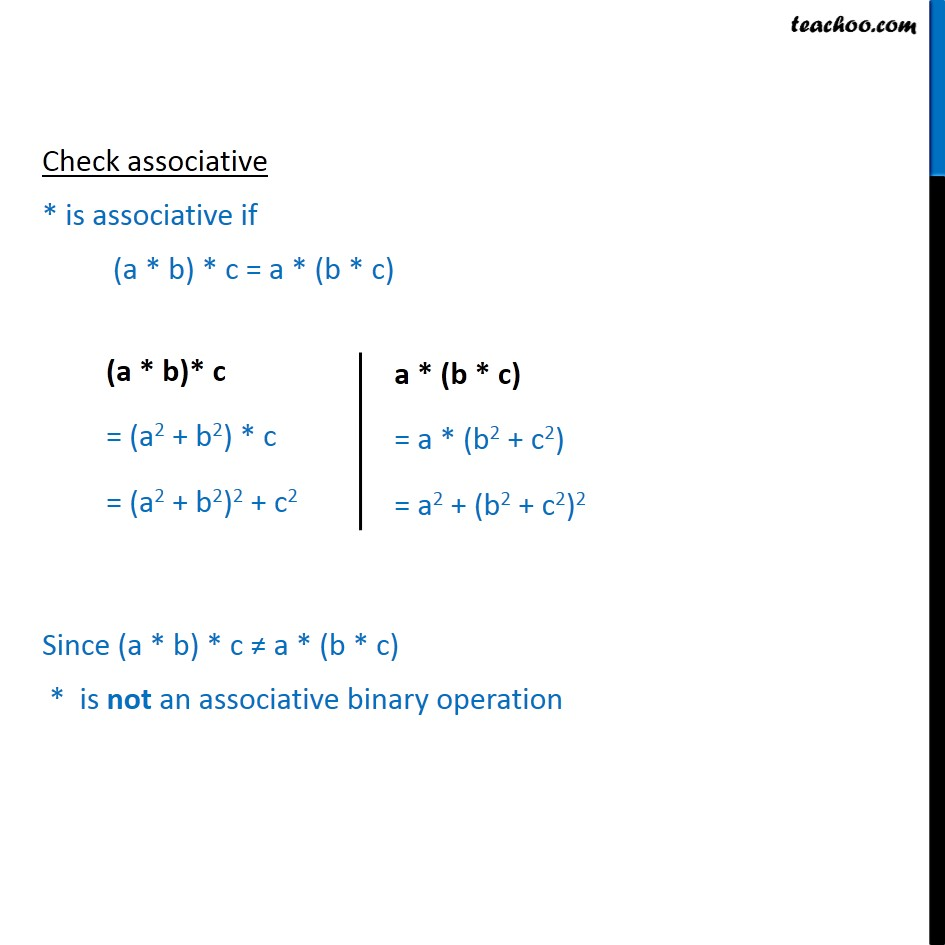Ex 1.4

Chapter 1 Class 12 Relation and Functions
Serial order wiseIntroducing your new favourite teacher - Teachoo Black, at only ₹83 per month

### Transcript

Ex 1.4, 9 Let * be a binary operation on the set Q of rational numbers as follows. Find which are associative and which are commutative : (ii) a * b = a2 + b2 Check commutative * is commutative if a * b = b * a Since a * b = b * a ∀ a, b ∈ Q * is commutative Check associative * is associative if (a * b) * c = a * (b * c) Since (a * b) * c ≠ a * (b * c) * is not an associative binary operation